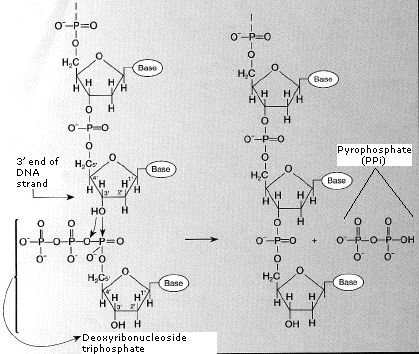# 2.13: Nucleotides

$$\newcommand{\vecs}{\overset { \rightharpoonup} {\mathbf{#1}} }$$ $$\newcommand{\vecd}{\overset{-\!-\!\rightharpoonup}{\vphantom{a}\smash {#1}}}$$$$\newcommand{\id}{\mathrm{id}}$$ $$\newcommand{\Span}{\mathrm{span}}$$ $$\newcommand{\kernel}{\mathrm{null}\,}$$ $$\newcommand{\range}{\mathrm{range}\,}$$ $$\newcommand{\RealPart}{\mathrm{Re}}$$ $$\newcommand{\ImaginaryPart}{\mathrm{Im}}$$ $$\newcommand{\Argument}{\mathrm{Arg}}$$ $$\newcommand{\norm}{\| #1 \|}$$ $$\newcommand{\inner}{\langle #1, #2 \rangle}$$ $$\newcommand{\Span}{\mathrm{span}}$$ $$\newcommand{\id}{\mathrm{id}}$$ $$\newcommand{\Span}{\mathrm{span}}$$ $$\newcommand{\kernel}{\mathrm{null}\,}$$ $$\newcommand{\range}{\mathrm{range}\,}$$ $$\newcommand{\RealPart}{\mathrm{Re}}$$ $$\newcommand{\ImaginaryPart}{\mathrm{Im}}$$ $$\newcommand{\Argument}{\mathrm{Arg}}$$ $$\newcommand{\norm}{\| #1 \|}$$ $$\newcommand{\inner}{\langle #1, #2 \rangle}$$ $$\newcommand{\Span}{\mathrm{span}}$$$$\newcommand{\AA}{\unicode[.8,0]{x212B}}$$

Nucleic acids are linear, unbranched polymers of nucleotides. Nucleotides consist of three parts.A five-carbon sugar (hence a pentose). Two kinds are found:

• deoxyribose, which has a hydrogen atom attached to its #2 carbon atom (designated 2'), and
• ribose, which has a hydroxyl group there.

Deoxyribose-containing nucleotides, the deoxyribonucleotides, are the monomers of deoxyribonucleic acids (DNA). Ribose-containing nucleotides, the ribonucleotides, are the monomers of ribonucleic acids (RNA).The Purines The Pyrimidines

A nitrogen-containing ring structure called a nucleobase (or simply a base). The nucleobase is attached to the 1' carbon atom of the pentose. In DNA, four different nucleobases are found:

• two purines, called adenine (A) and guanine (G)
• two pyrimidines, called thymine (T) and cytosine (C)

RNA contains:

• The same purines, adenine (A) and guanine (G).
• RNA also uses the pyrimidine cytosine (C), but instead of thymine, it uses the pyrimidine uracil (U).

## Nucleoside

The combination of a nucleobase and a pentose is called a nucleoside.One (as shown in the first figure), two, or three phosphate groups. These are attached to the 5' carbon atom of the pentose. The product in each case is called a nucleotide. Both DNA and RNA are assembled from nucleoside triphosphates.

• For DNA, these are dATP, dGTP, dCTP, and dTTP.
• For RNA, these are ATP, GTP, CTP, and UTP.

In both cases, as each nucleotide is attached, the second and third phosphates are removed.

Table 2.13.1: The nucleosides and their mono-, di-, and triphosphates
Nucleobase Nucleoside Nucleotides